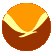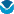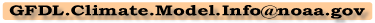# Geophysical FluidDynamics Laboratory

search gfdl:

### If you are using Navigator 4.x or Internet Explorer 4.x, this site will not render correctly!

gfdl's home page > products and services > data portal > deccen coupled climate model > CM2.X coupled climate model > faq

###What grid is used in the finite-volume atmospheric model component of CM2.1?

The finite-volume atmospheric model component of CM2.1 has 144 by 90 grids (or more precisely, "finite volumes"). This is the same number of points as in CM2.0, but the latitude grid locations differ in the two models.

Within the model itself, the winds are internally staggerred (C grid winds for the transport and D grid winds for defining the vorticity). All scalars (air/tracer mass and temperature) are defined as finite volume means. In the model output files available for download, winds have been averaged to the scalar point (i.e., center of the finite volume).

Given the resolution: `(nlon, nlat)=(144, 90)`, the bounding boxes `(xb, yb)` of the finite volumes are computed as

```        xb(i) = (i-1) * (360/nlon)           (i=1,2,... nlon+1)
```

Except the two poles (`j=1` and `j=nlat+1`),

```        yb(j) = -90 + (j-1.5)*180/(nlat-1)   (j=2,... nlat)
```

For `j=1`, `yb=-90`, and `yb=90` for `j=nlat+1`.

The centers of the finite volumes `(xc, yc)` are simply the average of the edges:

```        xc(i) = (xb(i) + xb(i+1)) / 2        (i=1,2, ...nlon)
yc(j) = (yb(j) + yb(j+1)) / 2        (j=1,2,... nlat)
```

#### Questions related to the GFDL CM2.x models may be directed to…